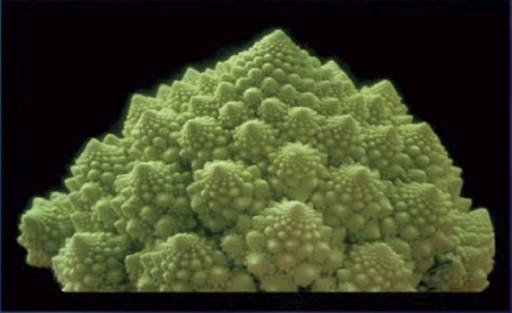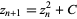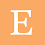### Fractals and Mandelbrot Set

While mathematics is in itself quite interesting and forms the basis of any modern day research, be it computational biology, machine learning or building complex structure, it can be quite a challenge to decide where to start.

That is why i decided to explore Fractals, thinking of it as a bridge between the nature and science. It brings in some really fascinating concepts which should be good enough for me as a gateway go deeper.

Fractals are in simple language never ending patterns which keep on repeating without an end, because fractals are never ending they have an infinite perimeter but finite area.

Since the patterns repeats indefinitely but if you draw a circle around the peremeter the area will remain finite.

It is like adding 1+0.1+0.01+0.001 and never making 2

This video explains the basic concept really well

Fractals are found everywhere nature in Trees, Rivers, Branching patterns, Hurricanes and Galaxies. It tries to bring order and understanding to the patterns that we see around us.While fractals are found in nature you can also generate them using mathematics using the Mandelbrot Set

Benoit Manderbrot, professor of Mathematical Sciences at Yale and the one who discovered fractals and hence the name.
It happens in the world of complex numbers which are plotted in a complex plane. The Mandelbrot set is the set obtained from the quadratic recurrence equationThe set of all complex values of  for which the point  does not escape to infinity under iteration of the function  is called the Mandelbrot set.

You can play around on these sites to explore more
Filled Julia set is like a generalization of mandelbrot set, so anything inside a julia set does not blow up and is stable. The Mandelbrot set is, in a sense, a dictionary of all quadratic Julia sets.

Julia set for C=0
Julia Set for C=1

The Manderbrot set is essentially a set of values of C for which the set is stable( its julia set ), the colors that we see define how stable the equation is black being very stable to blue, red being unstable. When we say its stable it means that the distance of the complex number from 0 is bounded and unstable means that the distance from 0 is unbounded or approaches infinity.

It is extremely fascinating how you are able to generate and visualize fractals, in fact it is like art being generated from mathematics with inspiration from nature. At the same time this is just scratching the surface and you can go really deep in this topic and its applications.

References:

1.This comment has been removed by the author.

2.This comment has been removed by the author.

3.Thank you for sharing this useful informative information. Math is a valuable tool for cultivating mental discipline since it encourages logical reasoning and mental rigor. Math has a lot of equations that can only be solved with the help of an expert maths tutor. Thank you for sharing valuable information about the mathematics topic.